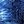Touch Light BulbWeird Circuits wrote 06/23/2020 at 09:39 • 4 min read • Like

https://www.instructables.com/id/Touch-Lamp-1/

https://www.instructables.com/id/Recycled-Components-Touch-Lamp/

The designs above show a low current LED driver. A typical LED needs only 10 mA. However, a typical 1.5 V light bulb needs 300 mA. I modified the circuit to drive higher current loads by adding an extra transistor.

You can see my circuit working in this video:

Step 1: Design the Circuit

https://www.instructables.com/id/Touch-Lamp-1/

You do not need 3 V power supply and you do not have to use Darlington pair transistors.

You design for 1.5 V power supply with two NPN and two PNP transistors (similar to: https://www.instructables.com/id/Touch-Lamp-1/) or 3 V power supply and use four NPN or four PNP transistors (similar to: https://www.instructables.com/id/Recycled-Components-Touch-Lamp/).

However, you must use a heat sink for the transistor that will drive the light bulb.

Determine Darlington pair transistor saturation voltage:

Vce3saturation = Vce3a + Vbe3b

= 0.2 V + 0.7 V = 0.9 V

Use of Darlington pair reduces the amount of components in the circuit but high saturation voltage (eg. 0.9 V instead of 0.2 V) increases the transistor power dissipation:

Pt = Vce * Ic

Calculate the minimum Q3 transistor current gain to ensure saturation:

Beta3Min = Ic3 / Ib3 = 0.3 A / ((Vs - Vce2 - Vbe3) / Rb3)

= 0.3 A / ((3 V - 0.2 V - 2 * 0.7 V) / 1000 ohms)

= 0.3 A / (1.4 V / 1000 ohms)

= 214.285714286

(this is a very low minimum gain for a Darlington pair transistor)

Calculate the minimum Q2 transistor current gain to ensure saturation:

Beta2Min = Ic2 / Ib2 = (((Vs - Vce2 - Vb3) / Rb3) + ((Vs - Vce2) / Rc2)) / ((Vs - Vce1 - Vbe2) / Rb2)

= (((3 V - 0.2 V - 2 * 0.7 V) / 1000 ohms) + ((3 V - 0.2 V) / 10,000 ohms)) / ((3 V - 0.2 V - 0.7 V) / 10,000)

= ((1.4 V / 1000 ohms) + (2.8 V / 10,000 ohms)) / (2.1 V / 10,000)

= 8

(a typical minimum gain for a general purpose transistor is 20)

Calculate the minimum Q1 transistor current gain to ensure saturation:

Beta1Min = Ic1 / Ib1 = (((Vs - Vce1 - Vbe2) / Rb2 + ((Vs - Vce1) / Rc1)) / ((Vs - Vbe1) / (Rb1 + Rhuman))

= (((3 V - 0.2 V - 0.7 V) / 10,000 ohms + ((3 V - 0.2 V) / 100,000 ohms)) / ((3 V - 0.7 V) / (100,000 ohms + 1,000,000 ohms))

= ((2.1 V / 10,000 ohms) + (2.8 V / 100,000 ohms)) / (2.3 V / 1,100,000 ohms)

= 113.826086957

If the resistance of a human is only 100 kohms then:

Beta1Min = ((2.1 V / 10,000 ohms) + (2.8 V / 100,000 ohms)) / (2.3 V / 200,000 ohms)

= 20.6956521739

Neither Q1 or Q2 transistors need to saturate for the circuit to work. They can just amplify the current. Transistor saturation does reduce the power dissipation. However, the first two transistors are not dissipating high currents and thus not high powers.

The Rs1/Cs1 and Rs2/Cs2 power supply low pass RC filters are used to filter power supply oscillations. Those oscillations could be due to 50 Hz/60 Hz main frequency noise or possible power supply oscillations that can occur even if you are powering the circuit with a 3 V battery source (two AA/AAA/C/D batteries in connected series). Those oscillations are caused by power source internal resistances.

Calculate the frequency of the low pass power supply filter:

fl1 = 1 / (2*pi*Rs1*Cs1) = 1 / (2*pi*100 ohms*(470*10^-6 F))

3.38627538493 Hz

You can try using Rs2 of 1 kohms and Cs2 of just 47 uF. The low pass frequency will be the same:

fl2 = 1 / (2*pi*Rs2*Cs2) = 1 / (2*pi*1,000 ohms*(47*10^-6 F))

3.38627538493 Hz

However, this will reduce the loading affect on the first power supply filter made from Rs1 and Cs1 resistors.

Step 2: Make the Circuit

I only used a soldering iron for the power Darlington pair transistor. I used TIP122 transistor.

You can see that I did not implement the second power supply filter. I used an old Soviet diode.

Step 3: Testing

The transistor seems to be fully saturated:

Discussions